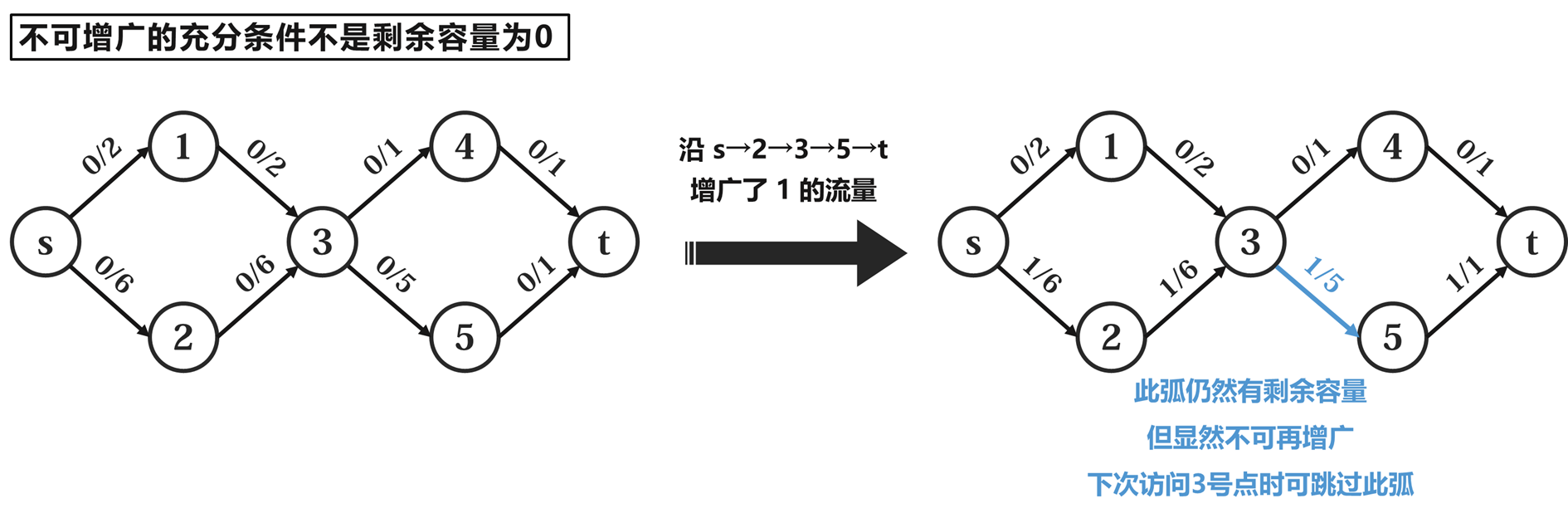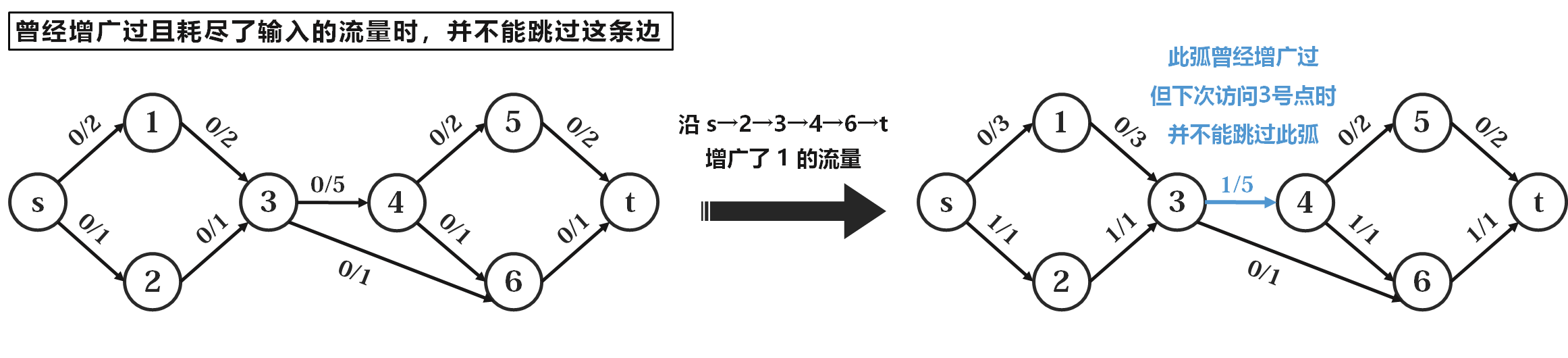#### 一、增广类算法

##### 算法实现

• $\text{FF}$：增广路算法的最朴素实现。在残量网络上不断 $\text{DFS}$ 找增广路，然后进行更新。对于整数容量网络，由于每次至少增广 $1$ 的流量，因此时间复杂度 $O(|max_{-}flow|)$。相对其他算法来说这是一个很糟糕的复杂度了，因此我们一般不使用此算法。

• $\text{EK(SAP, shortest augmenting path)}$：改进了一下 $\text{FF}$ 算法，用 $\text{BFS}$ 找增广路，并通过前驱数组进行更新。这样就保证每次都会按最短路（认为每条边距离都是单位 $1$）去增广。经证明可知其增广次数为 $O(VE)$ 的（详见算导。一个简要解释是，每条边成为限制当次增广流量的关键边的次数不会超过 $\text{V/2}$ 次，因为相邻两次成为关键边需要正着来一次和反悔来一次。记正着来之前 $dist[u]=a$，正着来一次后 $dist[v]$ 至少增成 $dist[u]+1=a+1$，再逆着来一次后 $dist[u]$ 就至少增成 $dist[v]+1=a+2$，所以每一对反悔操作都会让一条边的起点到源点距离至少增加 $2$，又因为距离最大不会超过点数，所以每条边最多成为 $\text{V/2}$ 次关键边，所以总共最多会有 $\text{E*V/2}$ 次关键边的出现，又因为每次增广至少消耗 $1$ 条关键边，所以最多增广 $\text{E*V/2}$ 次）。

所以总时间复杂度是 $O(VE^2)$ 的。

• $\text{Dinic}$：在 $\text{EK}$ 基础上再进行优化。还是不断 $\text{BFS}$ 找增广路，但与此同时额外进行标记深度操作（称为构造层次图）。且待 $\text{BFS}$ 完成发现汇点可达后，用 $\text{DFS}$ 进行沿最短路的增广，而不是直接用前驱数组进行沿最短路的增广。

易见，如果 $\text{Dinic}$ 也是每 $\text{BFS}$ 一次就 $\text{DFS}$ 一次，那还不如 $\text{EK}$ 直接用前驱数组来得方便。那 $\text{Dinic}$ 到底高在哪里呢？注意到我们的分层操作会把网络的拓扑结构保存起来，因此一次 $\text{BFS}$ 后其实是可以做多次增广的！因为 $\text{DFS}$ 不会破坏层次编号，所以我们在一次 $\text{BFS}$ 后可以一直 $\text{DFS}$ ，直到源汇不连通才进行下一次 $\text{BFS}$ 分层。这样效率就得到了提高。

此外，$\text{Dinic}$ 还有两个更强的优化：当前弧优化炸点优化，分别可以消除对不必要的弧和不必要的点的遍历。

• 当前弧优化：可以说搞懂如何正确地做这个优化后，整个 $\text{Dinic}$ 的运作过程就彻底弄明白了。

首先，什么叫当前弧优化？我们每次 $\text{DFS}$ 到某个点的时候，会按边的存储序依次访问其出边。如果能够确定其中前 $k$ 条边一定无法再增广，那么我这次就可以跳过这前 $k$ 条，从第 $k+1$ 条边进行这次增广。这就是当前弧优化。

那么如何确定某条边无法再增广了呢？是不是它剩余容量为 $0$ 才说明无法增广？不。 这里有一个非常显而易见的反例（$a/b$ 表示总容量为 $b$，使用了 $a$）：那怎么判断？可以这样：如果曾经有 $>0$ 的流量 $f{in}$ 放在了它的起点，但在经过增广后 $f{in}$ 并没有全部用完，那么就说明这条弧不可能再增广更多了。如上图，当沿着 $s\rightarrow2\rightarrow3\rightarrow5\rightarrow t$ 这条路增广的时候，曾经就有 $6$ 这么多流量放在了 $3\rightarrow5$ 的起点 $3$ 这里，然而最多只能沿这条弧流出 $1$。因此如果以后再访问到 $3$ 号点，$3\rightarrow5$ 这条弧就可以不看了。

【注意】 是曾经增广过且流量没用完才能跳过，而不是只要曾经增广过就能跳过！曾经增广过且耗尽了输入流量时，我们并不能跳过这条边，因为他可能还有增广的潜力（只是这次的流量输入不够所以只能增广这么多了，下次再来流量还可以再增广）。我们再举一个例子：如沿上图路径增广的时候，曾经有 $1$ 这么多流量放在了 $3\rightarrow4$ 的起点 $3$ 这里，然后流出了 $1$。显然，如果以后再访问到 $3$ 号点，我们并不能跳过 $3\rightarrow4$ 这条弧。比如下一次可以沿 $s\rightarrow1\rightarrow3\rightarrow4\rightarrow5\rightarrow t$ 这条路增广 $2$ 的流量。

所以这个地方一定要注意编程细节（可详见后续代码部分），否则稍不留神就会写错代码，使得当前弧优化变成当前弧劣化。

• 炸点优化：名字倒是挺凶的... qwq

其实就是在 $\text{DFS}$ 的过程中，如果遍历到了 $u$ 这个点的某条出边（记其指向 $v$ 这个点），且从这条边 $\text{DFS}$ 回来后发现一点流量都没有增广，就说明这棵子树的枝节全部都是盲端。那么就可以把 $v$ 为根的这棵子树全部炸掉（把这些点的层数编号设为一个负数，使得在下一次重新分层前他们就不会再被访问到了）

这就是 $\text{Dinic}$ 了，可以说它是求最大流最常用的算法了。后面会贴出其详细代码，其中的当前弧优化部分一定要仔细理清思绪。这样才能真正理顺此算法的流程，避免编写代码时翻车。

另外，经证明 $\text{Dinic}$ 的复杂度可达 $O(V^2E)$，面对一般的网络流问题已足矣。

• $\text{I SAP}$：
• ##### Dinic：
template <const bool directed, typename cint>
class Dinic
{
private:

static constexpr auto CINT_MAX = std::numeric_limits<cint>::max();

struct Ed   // 链式前向星。由于存在异或找反边的trick，使用下标式而不是指针式。（就算存指向反边的指针也不如异或快，实测）
{
int ne, v;
cint c;
} ed[ME];
int V, S, T;

int de[MV];
bool bfs()
{
static int q[MV];
int hd = 0, tl = 0;

memset(de, 0, sizeof(*de) * (V+2));
de[q[tl++]=S] = 1;
while (hd != tl)
{
const int u = q[hd++];
{
const int v = ed[i].v;
if (ed[i].c && !de[v])
{
de[q[tl++]=v] = de[u] + 1;
if (v == T)
return true;
}
}
}

return false;
}

cint dfs(const int u, cint in)  // 进dfs时，保证in一定 > 0
{
if (u == T)
return in;
cint out = 0;

/* 【当前弧优化】：跳过一定无法再增广的边。
什么时候可以认为一条边一定无法再增广了？就是曾经有>0的in流量放在了它的起点，但在经过增广后in流量并没有用完。这就说明它不可能再增广更多了。
因此for循环每进行一次（相当于榨干了一条边的增广潜力），我们就可以更新一次cur[u]，直到in用完或者已经遍历完u的一切出边了。
所以要注意最后一次循环到底是怎么退出的：
1. (in==0 && i!=0) || (in==0 && i==0)：
假设最后一次循环内这条边叫ed[i0]。ed[i0].ne==i1。那么ed[i0]可能是仍然能增广的（这次是因为in没了才没增广成功的，如果再给一些in，可能还可以增广）
那么退出for之后cur[u]应该是多少？显然应该是i0（因为ed[i0]可能还有潜力，跳过他就可能错过了，就失去了优化效果。）。
所以我们不能把for循环写成下面三种样子（他们是互相等价的）：（写成这样的话退出循环后cur[u]就会是i1了）
a) for (int i=cur[u]; i&&in; cur[u]=i=ed[i].ne)
b) for (int &i=cur[u]; i&&in; i=ed[i].ne)（和a写法完全等价）
c) for (int i=cur[u]; (cur[u]=i) && in; i=ed[i].ne)（和a写法相比只是在进入循环时多了一次没用的赋值）
但是这样可以：（刚好利用了&&的短路性质，使得for循环因为in==0退出时，cur[u]不会被更新成i1）
d) for (int i=cur[u]; in && (cur[u]=i); i=ed[i].ne)
或者我们把in==0时跳出的这句话给移出for的第二部分，移到for循环体内，那还是可以用以上三种写法的（特别是引用，这个最方便）。
2. in!=0 && i==0：
只有这种情况才可以把cur[u]更新成i2，且此时i2刚好是0。

所以到底怎么写当前弧优化呢？上面有一种利用&&短路性质的写法。除了这种，更常见的写法是在for循环体里面写。
那么写在哪？只要写在if外面都能起到及时更新而又不会在in==0时误更新的效果（不用担心写在for循环体最后会导致更新不及时。因为中间的递归过程里不可能再经过u这个点（因为一直是按dep的严格增序dfs的））
而最好不要写在if里面。不能说这条边没进if就不更新了。实际上如果这条边连再向下dfs都不行的话，更说明他无法再增广了。

但是这些在for里面写的写法有极为轻微的性能降低，因为他们绝对不会在循环结束的那次进行更新。
所以如果循环结束时in!=0而i==0，他们就没更新，而实际上这种情况是可以把cur[u]更新成i（也就是0）的。因为in流量还有剩余，说明u发出的所有边都一定不能再增广了

不过可以通过在最后炸点的时候把in!=0的点也炸掉来弥补这个微弱的性能降低
*/
for (int i=cur[u]; in&&(cur[u]=i); i=ed[i].ne)              // 【判in优化】：剪枝
{
if (ed[i].c>0 && de[ed[i].v]==de[u]+1)
{
const cint tp = dfs(ed[i].v, MIN(in, ed[i].c));     // 【多路增广优化】：一次bfs分层，多次增广。
in -= tp, out += tp, ed[i].c -= tp, ed[i^1].c += tp;
}
}

// 因为进函数时in必>0，所以退出函数前若in==0则out必!=0，而in!=0时out可能为0（没增广或者增广了0）可能不为0，out==0时in可能为0（in耗尽）也可能不为0（没耗尽）
// 所以if的两个条件并不是包含关系，因此不用担心某个条件是多余的
if (in || !out) // in不等于0就退出了for，说明u的所有出边都无法再增广；out==0则显然是增广失败的标志。
de[u] = -1; // 【炸点优化】：此路不通，剪掉盲枝
return out;
}

public:

void init(const int v)
{
V = v;
tot = 1;    // 不是2，如果tot是2那第一条边就是3了... 要让第一条边是非0偶数才行
}

void edd(const int u, const int v, const cint c = CINT_MAX) // 不填容量就是加无穷大容量
{
}

cint max_flow(const int s, const int t)
{
cint sum = 0;
S = s, T = t;
while (bfs())
{
sum += dfs(s, CINT_MAX);
}
return sum;
}
};


### 这篇文章有 3 个评论

1.test

2.小田田tql

3.榜一